# Catch up on the latest AI articles# Is LLM "Emergence" An Illusion?

3 main points
✔️ Validates emergence observed in large-scale language models
✔️ Suggests that LLM emergence may be an illusion created by evaluation measures
✔️ Successfully reproduces emergence that does not actually occur intentionally by using specific evaluation measures in non-LLM models

written by Rylan SchaefferBrando MirandaSanmi Koyejo
(Submitted on 28 Apr 2023 (v1), last revised 22 May 2023 (this version, v2))

Subjects: Artificial Intelligence (cs.AI); Machine Learning (cs.LG)code：The images used in this article are from the paper, the introductory slides, or were created based on them.

## Introduction

Emergence" refers to the occurrence of effects and phenomena that could not be seen in a single element when a large number of elements are assembled. In recent large-scale language models (LLMs), i.e., pre-trained decoders for transformers such as GPT that have a large number of parameters, many studies have observed that increasing the number of parameters enables solving tasks not seen in smaller models, or significantly increases accuracy. studies have observed that increasing the number of parameters also allows solving tasks that were not seen with smaller models, or significantly increases accuracy. These phenomena are often expressed by the sudden increase in scores when the number of parameters is increased in a graph where the horizontal axis represents the number of parameters on a logarithmic scale and the vertical axis represents the score of the evaluation index for a specific task (see the figure below).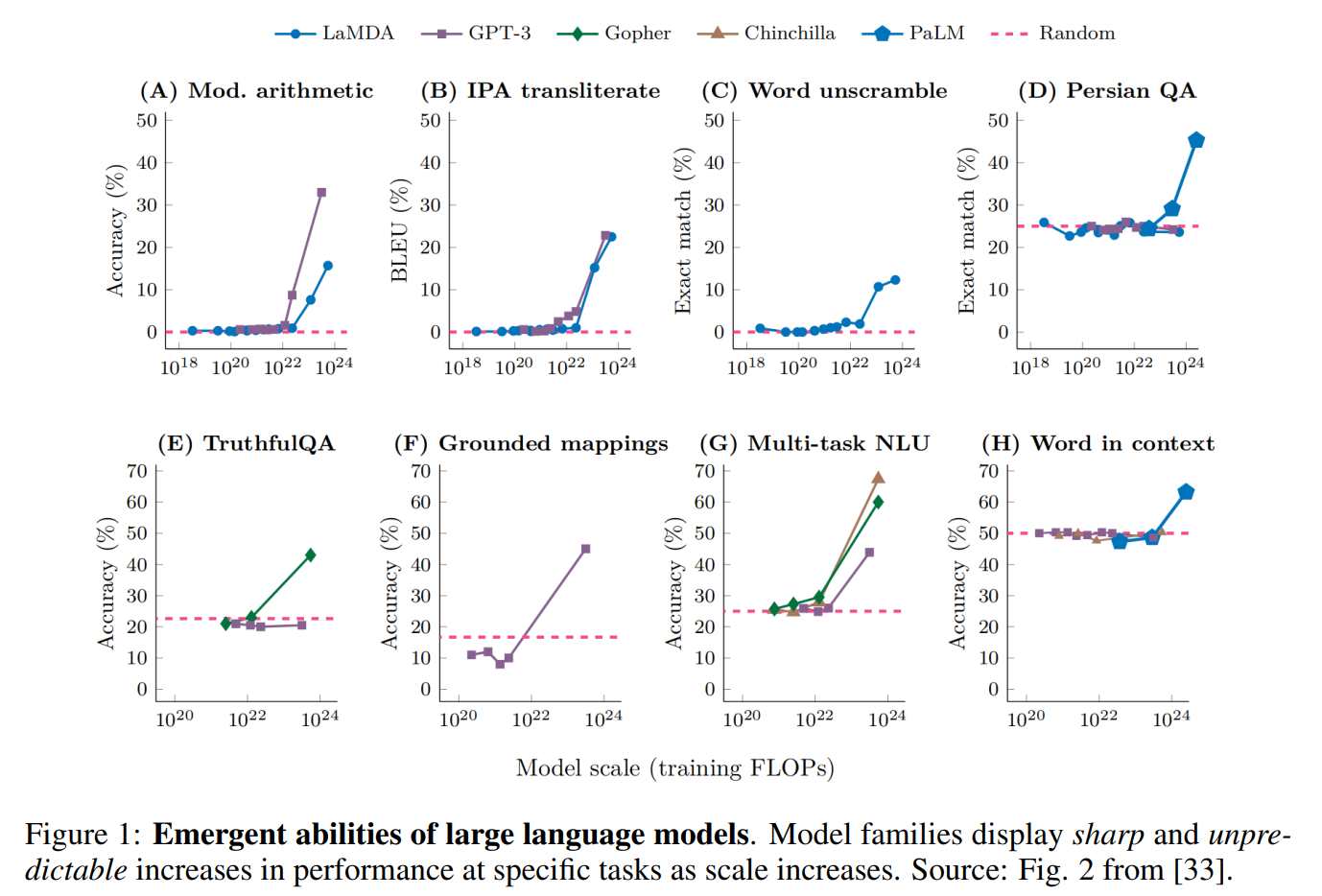However, this paper argues that the "emergence" of LLMs is a "phantom" that is seen through artificially selected metrics by researchers. This paper is one of them.

## The true nature of emergence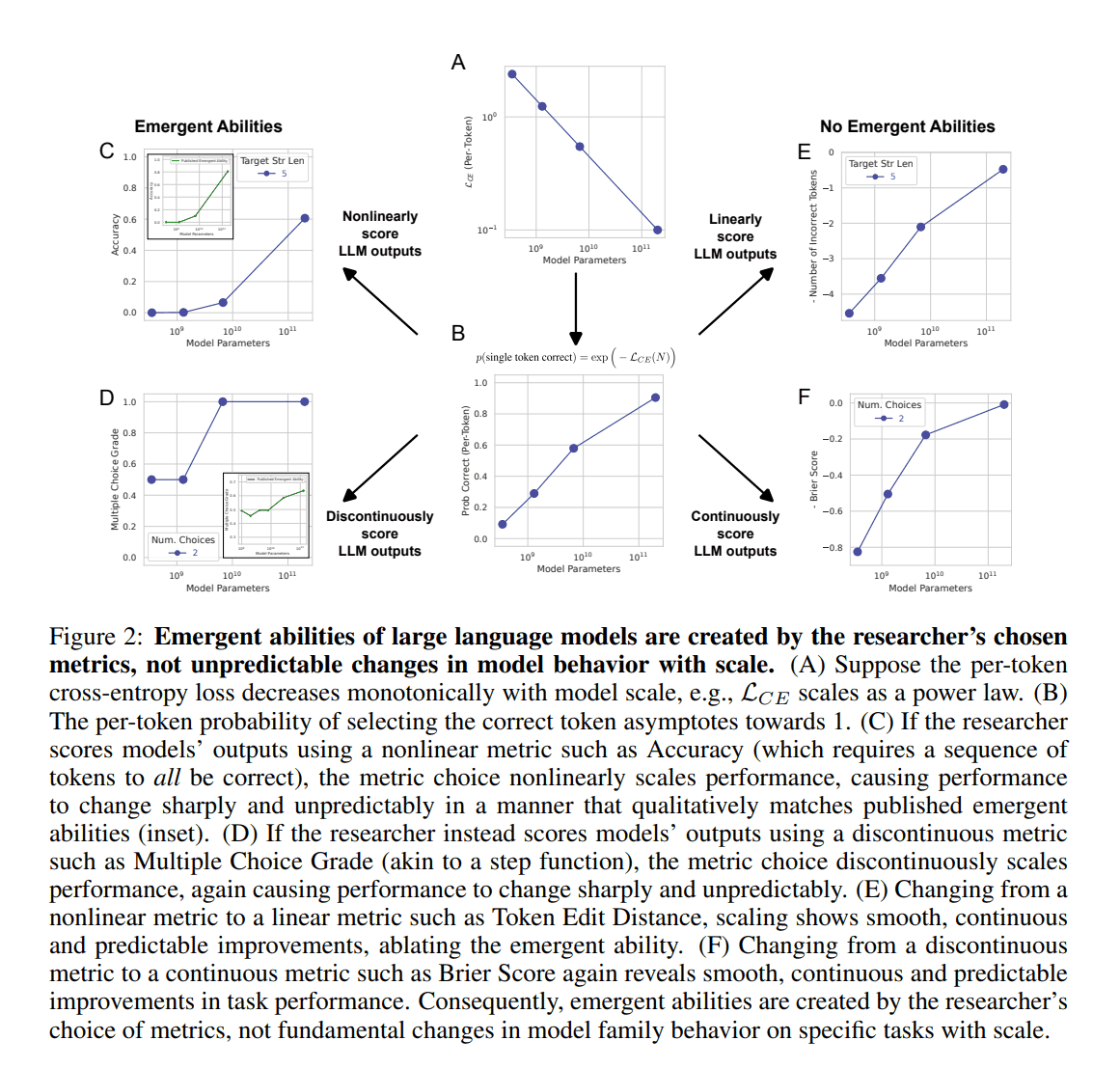First, we explain the relationship between the number of model parameters and prediction accuracy. It is well known that, in general, as the number of parameters is increased, the loss on test data decreases. This is the so-called scaling law. Assuming this law, the number of parameters is $N$, and the constants $c>0 and \alpha < 0$, the relationship between parameters and performance can be expressed as follows.

$$\mathcal{L}_{CE}(N) = \left(\frac{N}{c} \right)^\alpha$$

The $CE$ is the cross-entropy, commonly used as a loss function. This expression means that the higher the number of parameters, the lower the loss (see Fig. 2-A).

Letting $V$ be the set of assumed vocabulary (tokens), $p(v) \in \Delta^{|V|-1}$ be the unknown true probability for token $v$, and $\what{p}_N(v) \in \Delta^{|V|-1}$ be the prediction probability, the cross-entropy for each token is formulated as follows The cross-entropy for each token can be formulated as follows.

$$\mathcal{L}_{CE}(N) = - \sum_{v \in V} p(v)\mathrm{log} \, \hat{p}N(v)$$

However, in reality, in most cases, the true probability distribution of the lexical set is not known, so the one-hot distribution of the empirically observed tokens $v^*$ is approximated as follows.

$$\mathcal{L}_{CE}(N) = -\mathrm{log} \, \hat{p}N(v^*)$$

Based on the above, the probability of correct prediction for each token can be expressed as follows (see Fig. 2-B).
$$p(\text{single token correct}) = \exp(-\mathcal{L}_{CE}(N)) = \exp(-(N/c)^\alpha)$$

Let us consider a measure of whether each series of output tokens is in perfect agreement with the correct answer, such as the measure of the correctness of a solution to an addition of $L$-digit integers. When each output token is considered independent, the probability that the model's $L$-length prediction is correct (the percentage correct) is as follows

$$\operatorname{Accuracy}(N) \approx p_N(\text{single token correct})^{\text{num. of tokens}} = \exp \left(-(N/c)^\alpha \right)^L$$

The performance of this metric increases nonlinearly with increasing series length (see Fig. 2C). Plotting this on a logarithmic scale graph, it appears that sharp "emergence" has occurred. Now, let's change to a linear evaluation metric such as Token Edit Distance in the equation below.

$$\operatorname{Token Edit Distance}(N) \approx L(1-p_N(\text{single token correct})) = L(1-\exp(-(N/c)^\alpha))$$

When this was done, a smooth and continuous increase in performance appeared, as shown in Fig. 2E. Similarly, "emergence" disappeared when a discontinuous measure such as Multiple Choice Grade (Fig. 2D) was replaced by a continuous one such as Brier Score (Fig. 2F).

The authors summarize emergence as being explained by the following three elements

1. The researcher is using nonlinear or discontinuous evaluation metrics
2. Insufficient number of models (parameter variations) used in experiments
3. Insufficient test data

To be more specific, 1. emergence is an illusion shown by some of the evaluation indices, 2. on the horizontal axis of the result chart, a rapid change in accuracy can be avoided by preparing a more detailed model for each parameter and evaluating it, and 3. on the vertical axis of the result chart, performance stagnation around 0 can be eliminated by increasing test data and conducting more granular evaluations. The stagnation of performance around 0 can be eliminated by increasing the test data and conducting more granular evaluations.

## Analysis of observed emergence

In this section, we analyze how the results change when the evaluation index is changed for the emergence seen in existing LLMs computational tasks, etc.

The following hypotheses were formulated for the experiment, based on the three factors of emergence mentioned above.

1. Emergence disappears when the evaluation index is changed from nonlinear and discontinuous to linear and continuous
2. Increasing the test data and performing higher resolution evaluations results in a (presumably) linear increase in performance, even with nonlinear evaluation metrics.
3. Regardless of the evaluation metric, lengthening the tokens to be predicted has a significant impact on the change in performance (Accuracy is geometric, Token Edit Distance is linear)

The model uses InstructGPT and GPT-3. The problem is a 2-shot prediction of two-digit multiplication and four-digit addition.

### Disappearance of emergence by evaluation index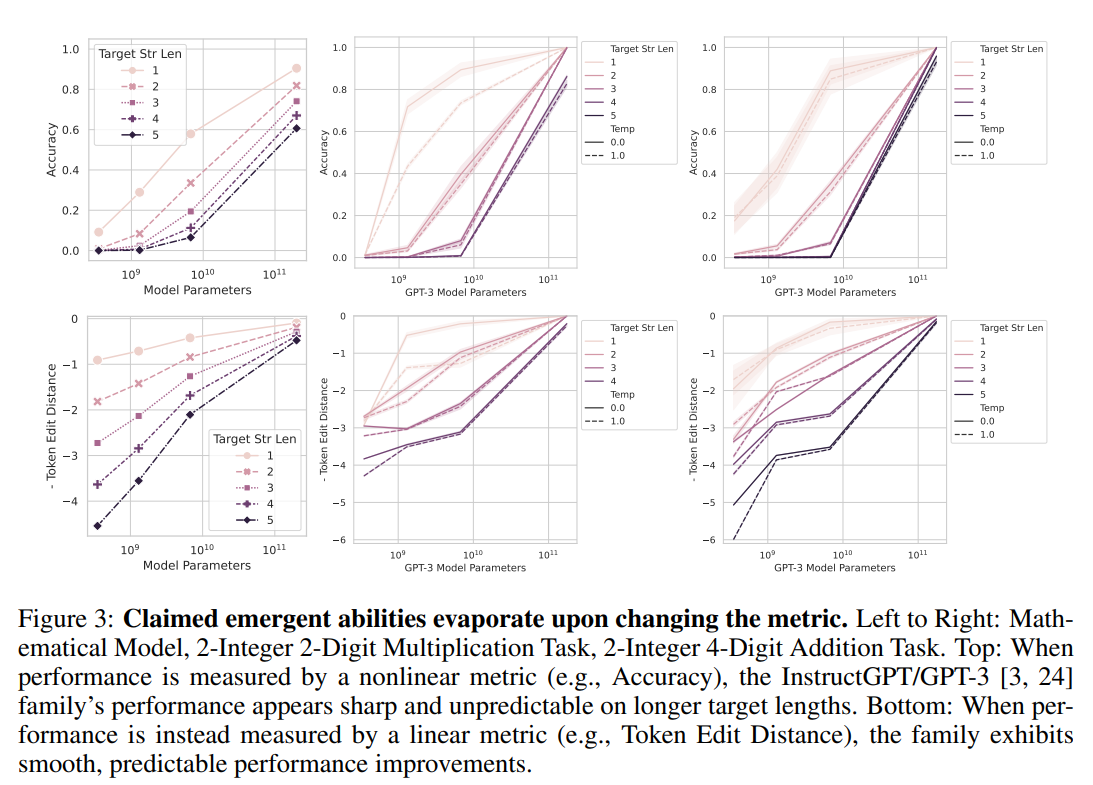The experimental results for the first hypothesis are shown in Fig. 3 above. When the evaluation index is "Accuracy" (perfect answer agreement) (upper part of the figure), we can see that the performance increases rapidly around the parameter $10^{10}$. On the other hand, when the evaluation indicator is changed to Token Edit Distance (lower part of the figure), the performance change seen with Accuracy disappears and a smooth performance change is observed.

### Emergence extinction through detailed parameter-by-parameter evaluation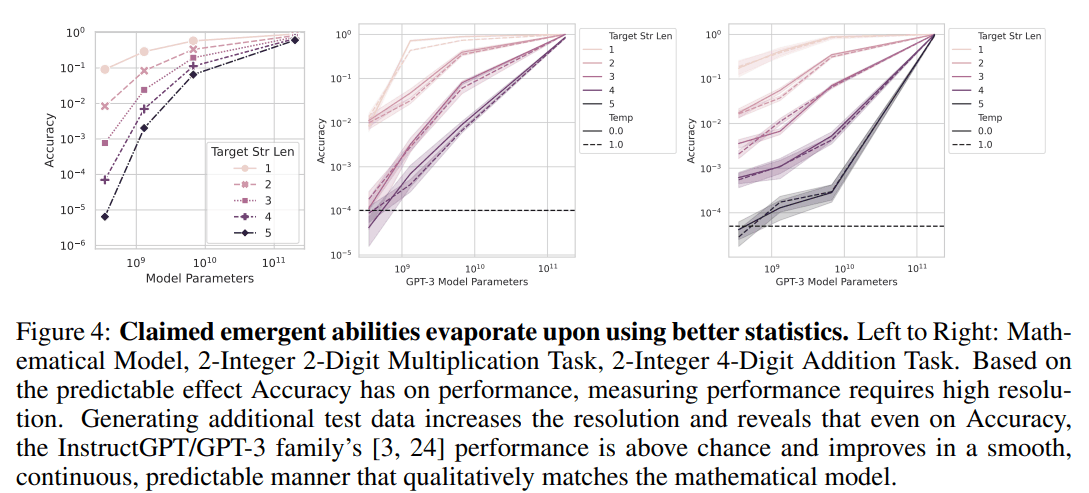Fig. 4 shows the experimental results for the aforementioned hypothesis regarding the test data. By increasing the test data, the accuracy can be calculated to finer (smaller) values.

In the upper half of Fig. 3, the small model seems to be stuck at 0 for a long time, and it looks as if it has no ability to respond to the target task. However, by increasing the test data and computing Accuracy to smaller values, we can see that there is in fact no lack of ability to respond, and that performance increases correspondingly as the parameters are increased.

In the experiments that confirmed the third hypothesis, it seems that as the target token length increases, performance declines geometrically with Accuracy and linearly with Token Edit Distance, but no figures or tables were provided in the paper.

## Meta-Analysis of Claimed Emergent Abilities

Due to various limitations, the experiments up to this point have been performed only with GPT-3.5/4. To show that emergent illusions are not observed only in a specific model, but depend on a set of evaluation indices, we examine how much each of the indices, called the BIG-bench, which is commonly used in the evaluation of LLMs, is illusory.

The Emergence Score is a measure of how likely a given metric is to produce emergent illusions. Let $y_i$ be the value of the target measure at the model scale $x_i \; (x_i < x_{i+1})$.

$$\operatorname{Emergence Score} \left(\left\{(x_n, y_n)\right\}_{n=1}^N \right) = \frac{\operatorname{sign}(\mathrm{argmax}_i y_i - \ mathrm{argmin}_i y_i(\mathrm{max_i}y_i - \mathrm{min}_i)}{\sqrt{\operatorname{Median}(\{ (y_i - y_{i-1})^2\} _i)}}$$

At first glance, this may seem complicated, but in essence, it indicates the degree to which the value of the evaluation index changes nonlinearly (i.e., the line becomes a "wiggly" curve) when the parameter is changed, and the larger the value, the stronger the change.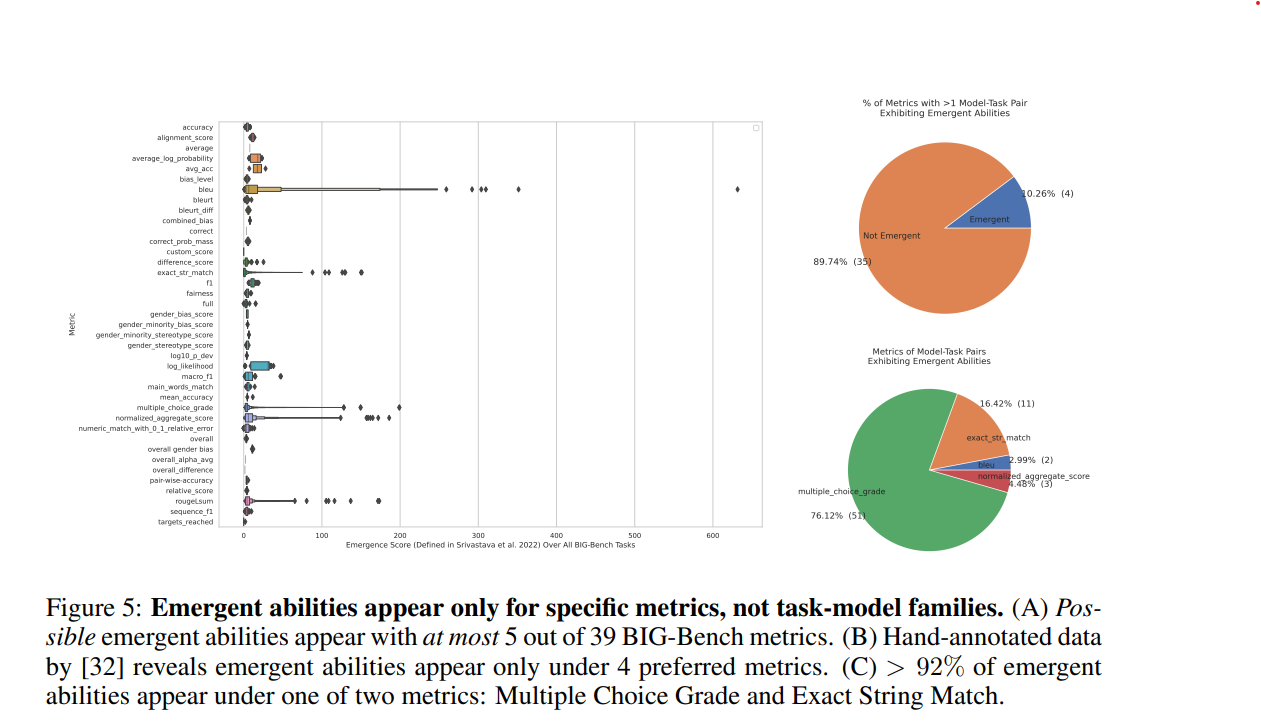Experimental results showed that the majority of the metrics in the BIG-Bench did not show emergent ability for task-model pairs; five of the 39 metrics showed high emergence scores, many of them nonlinear. Analysis of the task-model-rater triplet revealed that four out of 39 raters were emergent, with more than 92% of them being Multiple Choice Grade and Exact String Match. In short, only a small fraction of BIG-Bench's 39 metrics were nonlinear enough to show emergence.

We are also experimenting to see if emergence disappears when the evaluation index is changed within BIG-Bench. LaMDA was used as the model, and the results were analyzed by changing from Multiple Choice Grade to Brier Score. The results show that the nonlinear performance changes observed with Multiple Choice Grade are no longer observed with the Brier Score. This indicates that emergence is a function of the choice of the evaluation index.

## Inducing Emergent Abilities in Networks on Vision Tasks

The authors argue that the emergence of a model depends on a metric. Therefore, they predict that emergence can be "reproduced" by non-linguistic models. To prove this, they experiment with various neural nets (all-join, convolutional, and self-attention) to intentionally generate emergence.

### CNN on MNIST

LeNet, a CNN, is trained on MNIST, a dataset of handwritten digit images labeled with those digits, to solve the input handwritten digit classification task.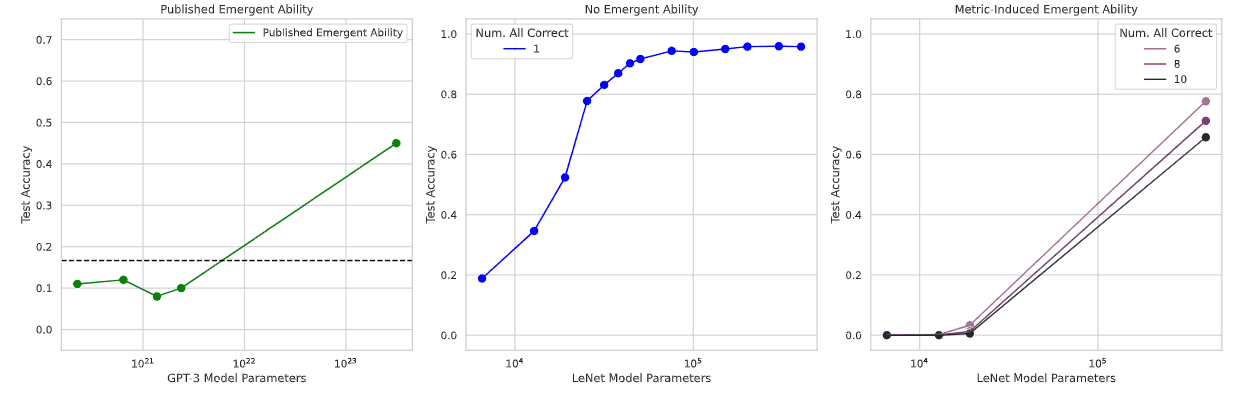The result is shown in the above figure. The middle graph, B, is Accuracy, which shows a smooth increase in accuracy as the parameter increases. On the other hand, the rightmost graph, C, uses an evaluation index called subset accuracy, which outputs 1 if all classes (in this case, numbers from 0 to 9) are correctly classified, and 0 otherwise (i.e., not a single error is allowed in each class classification). When the number of parameters is increased, a phenomenon is observed in which accuracy suddenly begins to increase from a point where it had previously remained near 0. This sudden increase is similar to the case of emergence already reported, as shown in the leftmost graph in Figure A. It can be said that we were able to reproduce emergence.

### Autoencoder on CIFAR100

A shallow auto-encoder with one hidden layer is trained to reconstruct the image with CIFAR100, defining a metric called Reconstruction that is likely to show emergence (illusion of emergence), and observing the change in value when the parameters are changed as before.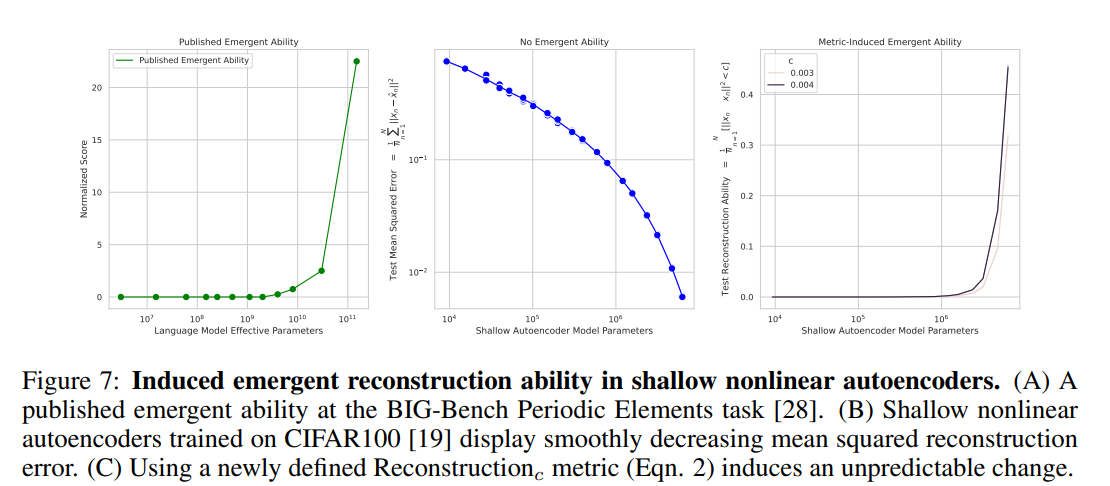The above chart shows the results. The middle graph, B, shows the change when Mean Square Error is used, and you can see a smooth decline. However, in Graph C, the leftmost graph showing the change when Reconstruction is used, it is observed that the state of almost no task ability suddenly began to show ability. This is very similar to the known case of emergence in Graph A, indicating that emergence is reproduced.

### Autoregressive Transformers on Omniglot

The images embedded in one convolution layer are classified by a decoder-only Transformer, which, like a CNN, attempts to reproduce emergence by classifying L-length strings and using a subset accuracy metric that gives 1 if all the strings are correct and 0 otherwise.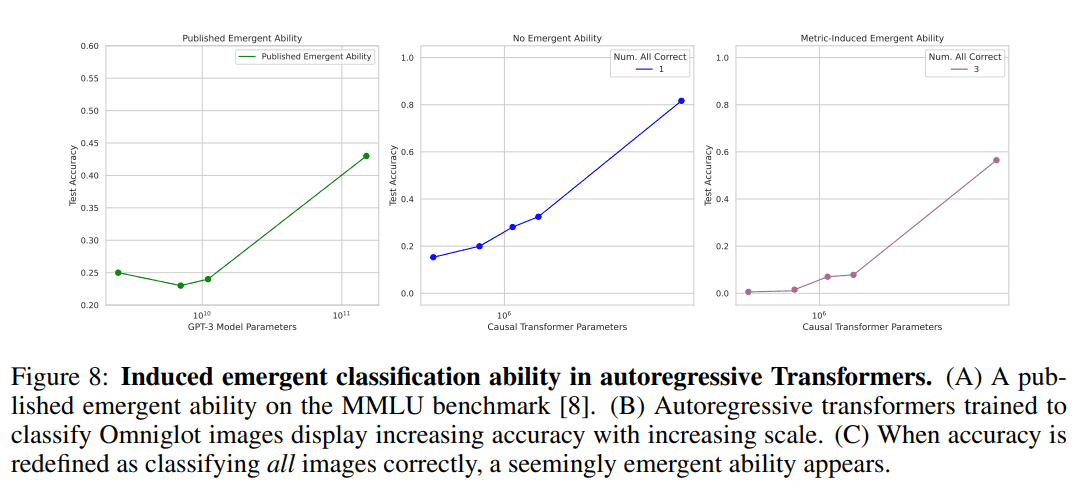While the performance of the normal accuracies in the middle increases linearly with increasing parameters, the results using the subset accuracies on the rightmost panel are similar to the leftmost reported case of emergence on the MMLU benchmark, indicating that emergence is artificially reproduced. The results are similar to the leftmost reported case of emergence on the MMLU benchmark, indicating that emergence is artificially reproduced.

From the above experiments using multiple architectures, we found that it is possible to make it appear as if emergence is occurring by artificially selecting evaluation indicators, regardless of the size of the model.

## Conclusion

The paper suggests that, with respect to the emergence of language models, what is seen is actually an illusion, depending on the choice of the evaluation index.

However, the authors state that this does not mean that there are no capabilities gained through emergence in the language model, but merely that emergence as currently accepted may not actually be the case.

I think this study shows that we should be very careful in selecting evaluation indices for any experiment.

If you have any suggestions for improvement of the content of the article,